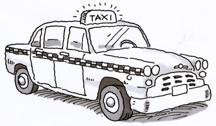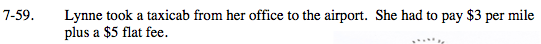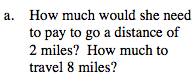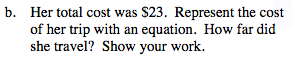### Home > MC2 > Chapter 7 > Lesson 7.1.6 > Problem7-59

7-59.
1. Lynne took a taxicab from her office to the airport. She had to pay $3 per mile plus a$5 flat fee. Homework Help ✎1. How much would she need to pay to go a distance of 2 miles? How much to travel 8 miles?

2. Her total cost was $23. Represent the cost of her trip with an equation. How far did she travel? Show your work.If each mile costs$3, 2 miles would cost $11.$6 plus the flat fee of $5. How much would 8 miles cost? 2 miles :$11
8 miles: $29If we let a variable represent the miles traveled, we can create an expression to represent the cost for her transportation. Since each mile costs$3, this can be represented as $3(x). Because she had to also pay a flat fee, this can be represented as a constant,$5.

$3(x) +$5

$3(x) +$5 = \$23
How many miles did she travel?